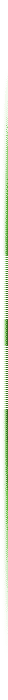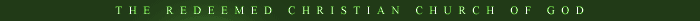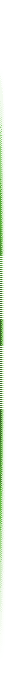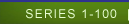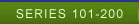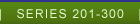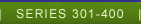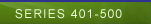LESSON 201- 225 Select Lesson Lesson 201 Lesson 202 Lesson 203 Lesson 204 Lesson 205 Lesson 206 Lesson 207 Lesson 208 Lesson 209 Lesson 210 Lesson 211 Lesson 212 Lesson 213 Lesson 214 Lesson 215 Lesson 216 Lesson 217 Lesson 218 Lesson 219 Lesson 220 Lesson 221 Lesson 222 Lesson 223 Lesson 224 Lesson 225 LESSON 226- 250 Select Lesson Lesson 226 Lesson 227 Lesson 228 Lesson 229 Lesson 230 Lesson 231 Lesson 232 Lesson 233 Lesson 234 Lesson 235 Lesson 236 Lesson 237 Lesson 238 Lesson 239 Lesson 240 Lesson 241 Lesson 242 Lesson 243 Lesson 244 Lesson 245 Lesson 246 Lesson 247 Lesson 248 Lesson 249 Lesson 250 LESSON 251-275 Select Lesson Lesson 251 Lesson 252 Lesson 253 Lesson 254 Lesson 255 Lesson 256 Lesson 257 Lesson 258 Lesson 259 Lesson 260 Lesson 261 Lesson 262 Lesson 263 Lesson 264 Lesson 265 Lesson 266 Lesson 267 Lesson 268 Lesson 269 Lesson 270 Lesson 271 Lesson 272 Lesson 273 Lesson 274 Lesson 275 LESSON 275-300 Select Lesson Lesson 276 Lesson 277 Lesson 278 Lesson 279 Lesson 280 Lesson 281 Lesson 282 Lesson 283 Lesson 284 Lesson 285 Lesson 286 Lesson 287 Lesson 288 Lesson 289 Lesson 290 Lesson 291 Lesson 292 Lesson 293 Lesson 294 Lesson 295 Lesson 296 Lesson 297 Lesson 298 Lesson 299 Lesson 300DIGGING DEEP NO 201 PURE GOLD INTRODUCTION: When God told Moses to build Him a tabernacle, He insisted that every major vessel within it must be made of pure gold, Ex. 37: 2,6, 16,17, 23, 24. Now, we are the temple of the Holy Spirit and our God has not changed and He has not lowered His standards. He wants us to be pure as gold. May the Lord teach us tonight, in Jesus Name, Amen. 1. THERE IS NO ROOM FOR IMPERFECTION IN GOD'S TEMPLE Our God is perfect and we are commanded to be perfect too. Mat 5:48; Eph. 5:25-27; Jam. 1:27; 11 Pet. 3:14; Rev. 21: 27 In fact, unless we are, we can have no part in the body of Christ because there is no room for anything that defiles in haven 1 pet. 1 L15- 16; 1John. 1:5. God is pure Light and so we must be since we are His children must be like their father. Matt. 5: 14; 1 John 3:6-9; Jam. 1: 17; John 19:4; 1 John 2:28-29. 2. IF GOD LOVES YOU, HE WILL MAKE YOU PERFECT. If God loves you and is determined to get you to heaven, then He will make you perfect one way or the other. It could be through suffering, chastening, the rod of correction, the refining fire or if you so desire, through His word. Heb. 2:9-10; Heb. 5;8-9; Jam. 1:2-4; 1 pet. 5: 10; Heb. 12:5-11; pro. 22:15; Mal. 3:2-4; Zec. 13:8-9; 1 pet. 1:3-7; Heb.13:20-21; 11 Tim. 3:16-17; 11 Cor. 13:11; Eph. 4:13; Col. 1:28; 1 pet. 5:6; Deu. 8:2; 11 Cor. 12: 21; Heb. 6:1.